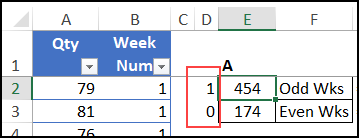## Excel Formula to Sum for Odd or Even Weeks

Written by Debra Dalgleish from Contextures Blog

Here’s an Excel formula challenge for you, based on an email question that someone sent to me. They wanted help with an Excel formula to sum for odd and even weeks. See my solution, and let me know how you’d solve the problem.

### Why Sum Odd or Even Weeks?

Have you ever needed to add numbers, based on odd and even numbers in another column?

In this example, the person worked in a factory, where there are 2 work crews – Crew A and Crew B. They need to compare the production quantities for the 2 work crews.

Here’s a simplified version of the production data, which we’ll use for this challenge. As you can see, the crew name is not in the data.

This is the morning data from the first 4 weeks of the year.### Identify the Crews

Even though the crew name is not listed, we can use the week numbers to identify which crew was working.

• Crew A works mornings on odd-numbered weeks
• Crew B works mornings on even-numbered weeks

The production data doesn’t show the crew name, but we can total the odd or even week data, to get each crew’s total quantity.

### Formula Challenge

Your challenge is to create a formula to calculate the total quantities for odd and even weeks

You can type the data in a blank workbook, or download my sample file with the challenge data and all the solutions that are shown below.

You can see my solution in the next section, and below that, you’ll see solutions from my weekly Excel newsletter readers.

What pros and cons can you see in the other people’s solutions?

If you found a different solution than the ones shown here, let me know in the comment section.

### My Solution

Here’s my formula to sum for odd or even weeks. I used the SUMPRODUCT function, combined with ISODD and ISEVEN.

I put this formula in cell E2, to total the odd weeks:

• =SUMPRODUCT((ISODD(–(\$B\$2:\$B\$11))) *(\$A\$2:\$A\$11))

And here’s the formula in cell E3, to total the even weeks:

• =SUMPRODUCT((ISEVEN(–(\$B\$2:\$B\$11))) *(\$A\$2:\$A\$11))#### How It Works

Here’s how my formula works:

• The ISODD and ISEVEN functions return TRUE or FALSE
• The two minus signs (double unary) convert those T/F values to numbers (-1 or 0)
• Those results are multiplied by the Qty amounts
• The SUMPRODUCT function returns the total of all those multiplications.

There are more Sumproduct examples on my Contextures website.

### Other Solutions

Next, here are some of the formulas that newsletter readers sent to me. You can get the data, and see all of the solutions in the Formula Challenge sample file.

#### MOD Function

Many of the solutions used the MOD function, to check if the week numbers were odd or even.

• The MOD function returns the remainder when you divide the first number (week number) by the second number (2)
• If you divide an even number by 2, the remainder will be zero
• If you divide an odd number by 2 the remainder will be 1

For example, to find the total for odd weeks:

=SUMPRODUCT((MOD(\$B\$2:\$B\$11,2)=1)*\$A\$2:\$A\$11)

### SUM or SUMPRODUCT

All the solutions used SUM or SUMPRODUCT to calculate the grand total.

For example, to find the total for even weeks:

=SUM(MOD(\$B\$2:\$B\$11-1,2)*\$A\$2:\$A\$11)

NOTE: Array Formulas

• In older versions of Excel, you’ll need to array-enter the SUM  formulas, with Ctrl+Shift+Enter.
• If your version of Excel has Dynamic Array formulas, you won’t need to do that — just press Enter.

#### FILTER Function

Two of the solutions use the new FILTER function. It filters a set of numbers, based on a rule.

=SUM(FILTER(\$A\$2:\$A\$11, MOD(\$B\$2:\$B\$11,2)))

In these solutions, the quantities were returned, based on using the MOD function in the week number column.

You can use these solutions if your version of Excel has Dynamic Array formulas.

### Hard-Coded Values

In some solutions, there were hard-coded values. For example, the Odd week formulas used a 1, and the Even week formulas used a zero.

• =SUMPRODUCT((MOD(\$B\$2:\$B\$11,2)=1) *\$A\$2:\$A\$11)
• =SUMPRODUCT((MOD(\$B\$2:\$B\$11,2)=0) *\$A\$2:\$A\$11)

You could put those values in adjacent cells, and refer to those cells in the formula.

=SUMPRODUCT((MOD(\$B\$2:\$B\$11,2)=D2)*\$A\$2:\$A\$11)Then, drag down, to use the same formula in both calculations. I like to use consistent formulas wherever possible!

### Hard-Coded Array

One solution used hard-coded arrays of odd and even numbers.

• =SUM(SUMIFS(\$A\$2:\$A\$11,\$B\$2:\$B\$11,{1,3}))
• =SUM(SUMIFS(\$A\$2:\$A\$11,\$B\$2:\$B\$11,{2,4}))

That worked alright in this small sample, with only 4 work weeks, but you’d need a flexible solution for larger samples.

### Helper Columns

A few people used one or two helper columns in their solutions.  You can see those in the sample file, and I’ve colour coded the columns, to match the solutions in which they’re used.Helper cells or columns are useful in some situations, allowing you to break a complex formula into smaller sections that are easier to understand or troubleshoot.

But in these solutions, you’d need formulas in every data row, instead of a just 2 formulas at the top of the worksheet.

That’s not too bad in a small file like this one, but could really slow things down if you’re working with thousands of rows of data.

### More Sum Examples

There are more How to Sum examples on my Contextures website.

And remember, you can get challenge data, and see all of the solutions in the Formula Challenge sample file.

___________________

### Excel Formula to Sum for Odd or Even Weeks___________________

Excel Formula to Sum for Odd or Even Weeks is a post from Contextures Blog and is not allowed to be copied to other sites